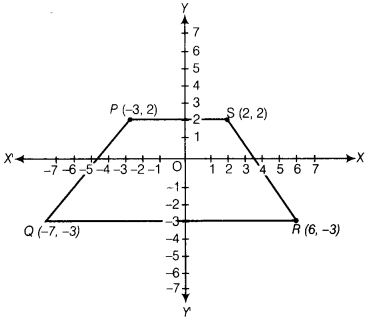# Plot the following points and write the name of the figure obtained by joining

Plot the following points and write the name of the figure obtained by joining, them in order.
P(-3,2),Q(-7, -3), R(6, -3), S(2,2)

Plotting the given points on the graph paper,Hence, we have the figure obtained as trapezium PQRS.